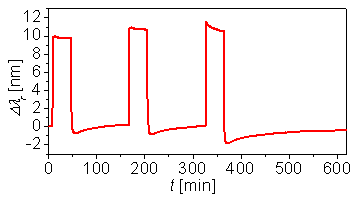Home News About Me Ph. D. Thesis Abstract Table of Contents 1. Introduction 2. Theory  Fundamentals of the Multivariate Data Analysis 3. Theory  Quantification of the Refrigerants R22 and R134a: Part I 4. Experiments, Setups and Data Sets 5. Results  Kinetic Measurements 5.1. Static Sensor Measurements 5.2. Time-resolved Sensor Measurements 5.3. Makrolon  A Polymer for Time-resolved Measurements 5.3.1. General Properties of Makrolon 5.3.2. Time-resolved Measurements 5.3.3. Thickness of the Sensitive Layer 5.3.4. Influence of the Carrier Gas 5.4. Conclusions 6. Results  Multivariate Calibrations 7. Results  Genetic Algorithm Framework 8. Results  Growing Neural Network Framework 9. Results  All Data Sets 10. Results  Various Aspects of the Frameworks and Measurements 11. Summary and Outlook 12. References 13. Acknowledgements Publications Research Tutorials Downloads and Links Contact Search Site Map Print this Page## 5.3.   Makrolon  A Polymer for Time-resolved Measurements

### 5.3.1.   General Properties of Makrolon

The time-resolved measurements of the SPR and RIfS setups are based on sensitive layers, which were prepared using a polycarbonate polymer. This polymer is commercially available as Makrolon M2400 from Bayer AG, Leverkusen, and will be further referred to as Makrolon. The structure of the monomer unit is shown in figure 17. The interesting property of Makrolon is the microporous structure of the glassy polymer. According to , the size of these pores follows a distribution with a mean pore size of 0.1 nm3 determined by the use of the positron annihilation lifetime spectroscopy (PALS). The PALS detects the decay of positrons and exploits the effect that the positrons are more probably present in the pores than in the polymer matrix due to the lower electron density in the pores ,.figure 17:  Structure of the monomer unit of the polymer Makrolon M2400.

In figure 18, the shift of the SPR wavelength is shown while the device was exposed to an alternating sequence of synthetic air and relative saturation pressures of methanol of 0.31, 0.62 and 0.80. At these high concentration levels, the sensor signal increases rapidly at the beginning of the analyte exposure meaning that the refractive index of the sensitive layer increases. This increase of the refractive index can only be explained by methanol (nD20=1.329) sorbing into the micropores of the polymer and replacing air (nD20=1) in these polymers as the sorption of methanol into the polycarbonate matrix (nD20=1.58) would decrease the refractive index of the sensitive layer. The molecules of methanol have less volume (0.068 nm3) than the mean size of the pores of Makrolon and therefore can easily sorb into the micropores.figure 18:  SPR sensor response of the SPR device when exposed to different relative saturation pressures of methanol of 0.31, 0.62 and 0.80 and alternating to synthetic air.

During a long-term exposure of Makrolon to methanol (see figure 18), the refractive index decreases. This decrease reinforces with higher concentrations of methanol and can be explained by two effects. The first effect is based on an expansion of the micropores of the polycarbonate matrix when these micropores are occupied by analyte molecules resulting in a decrease of the refractive index of the sensitive layer. When the exposure to methanol stops the refractive index decreases rapidly as the methanol molecules quickly desorb. After the exposure to high concentrations of methanol, the refractive index decreases below the initial value, as the pores and with it the Makrolon matrix are still expanded. The initial refractive index is gradually reached while the Makrolon layer is shrinking to the initial thickness. The expansion of the Makrolon matrix can only be detected when exposed to analyte for a long time. This effect allows the modification of the kinetics of sorption and desorption for bigger molecules and is exploited in section 5.3.4. The second effect is an unspecific sorption of the analyte into the polymer matrix besides of the specific sorption into the pores resulting in a swelling of the matrix. As the analyte has a lower refractive index than the polymer matrix, the shift of the SPR wavelength decreases. This unspecific sorption is a Henry type sorption (see section 3.2) whereas the sorption into the micropores can be considered as a Langmuir sorption (see section 3.2). In figure 19 the isothermal calibration curve for the sorption of methanol into Makrolon is shown. The curve shows the typical shape of the Langmuir sorption and hardly any portion of Henry sorption can be detected whereas the isothermal calibration curves of R22 and R134a show a significant Henry sorption (see figure 7 in section 3.2 and additionally the discussion in section 3.2). At the moment, further research is done by combining measurements of an ellispometric device, an SPR device and a RIfS device for distinguishing the sorption into the pores from the sorption into the matrix and for distinguishing the long-term expansion of the matrix from the swelling due to analyte sorption. First experiments allow following preliminary conclusions:

• The Henry sorption plays only a role if the analyte molecules are so big that a specific Langmuir sorption into the pores is not possible or very slow, whereby the total amount of the Henry sorption is always very low due to the high glass temperature of Makrolon.
• For exposure times less than 30 minutes, the expansion of the polymer matrix can be neglected.
• The sorption of analyte is reversible and the layer is highly stable. For example, the measurements, which are partly shown in figure 18, had a mean drift of 5.3*10-4 nm/h, which corresponds to only 0.01 % of the maximum sensor response.figure 19:  Isothermal calibration curve after 30 minutes of exposure to different concen­trations of methanol. The standard deviations of 3 measurements are represented by the error bars.

 Page 81 © Frank Dieterle, 03.03.2019